Скачать презентацию EXAMPLE 3 Make a table for a function

bb0352cdbcd451e5c0abc0d95ed29799.ppt

• Количество слайдов: 7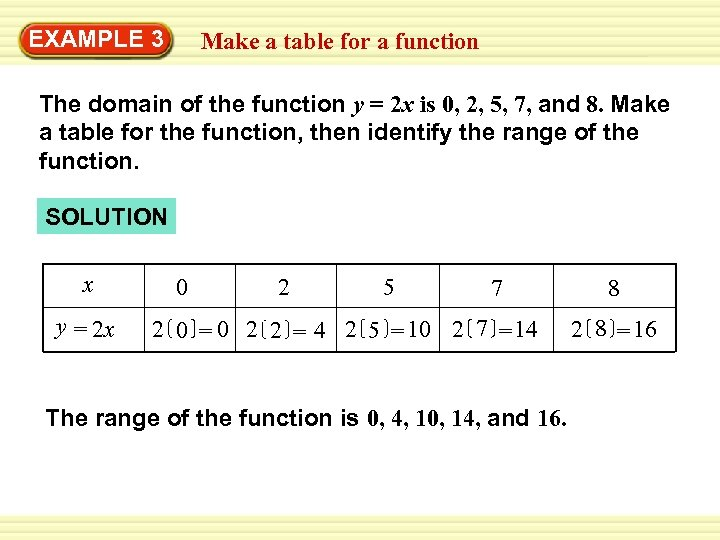EXAMPLE 3 Make a table for a function The domain of the function y = 2 x is 0, 2, 5, 7, and 8. Make a table for the function, then identify the range of the function. SOLUTION x y = 2 x 0 2 5 7 2 0 = 0 2 2 = 4 2 5 = 10 2 7 = 14 The range of the function is 0, 4, 10, 14, and 16. 8 2 8 = 16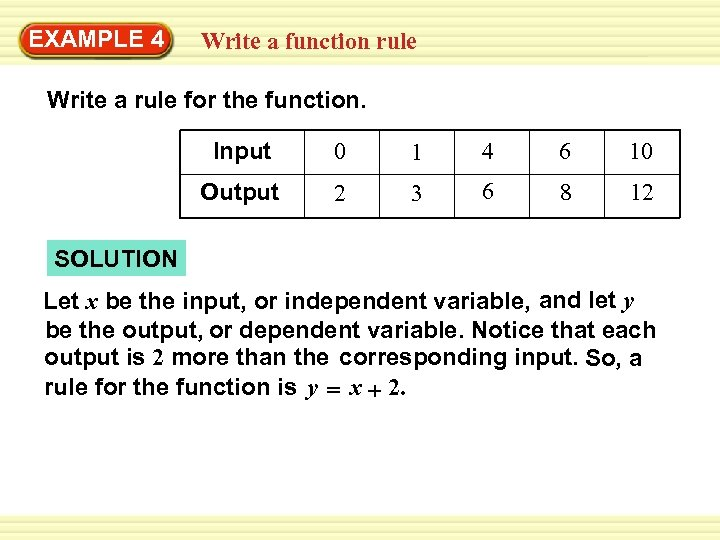EXAMPLE 4 Write a function rule Write a rule for the function. Input 0 1 4 6 10 Output 2 3 6 8 12 SOLUTION Let x be the input, or independent variable, and let y be the output, or dependent variable. Notice that each output is 2 more than the corresponding input. So, a rule for the function is y = x + 2.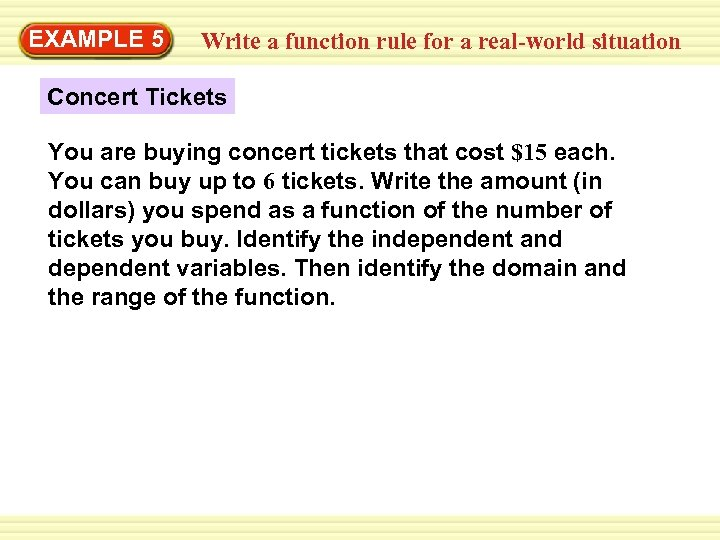EXAMPLE 5 Write a function rule for a real-world situation Concert Tickets You are buying concert tickets that cost \$15 each. You can buy up to 6 tickets. Write the amount (in dollars) you spend as a function of the number of tickets you buy. Identify the independent and dependent variables. Then identify the domain and the range of the function.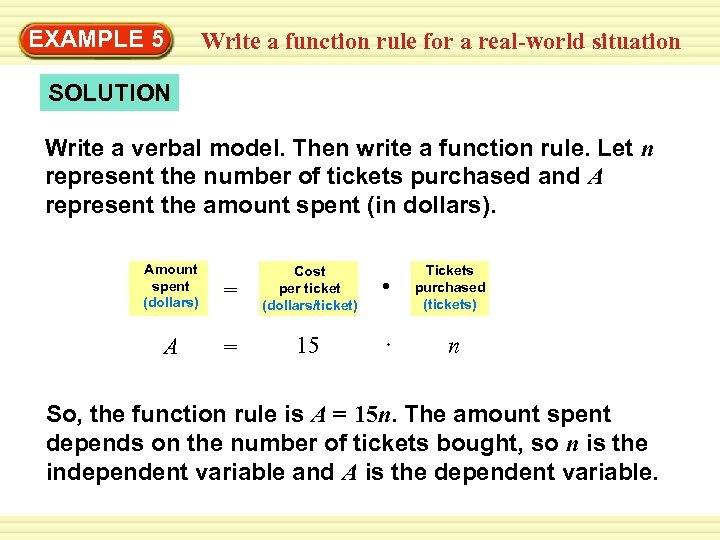EXAMPLE 5 Write a function rule for a real-world situation SOLUTION Write a verbal model. Then write a function rule. Let n represent the number of tickets purchased and A represent the amount spent (in dollars). Amount spent (dollars) = Cost per ticket (dollars/ticket) A = 15 • Tickets purchased (tickets) n So, the function rule is A = 15 n. The amount spent depends on the number of tickets bought, so n is the independent variable and A is the dependent variable.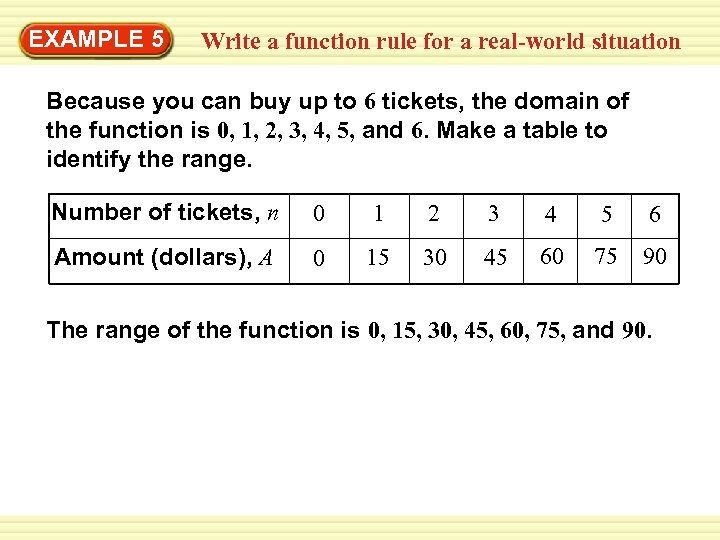EXAMPLE 5 Write a function rule for a real-world situation Because you can buy up to 6 tickets, the domain of the function is 0, 1, 2, 3, 4, 5, and 6. Make a table to identify the range. Number of tickets, n 0 1 2 3 4 5 6 Amount (dollars), A 0 15 30 45 60 75 90 The range of the function is 0, 15, 30, 45, 60, 75, and 90.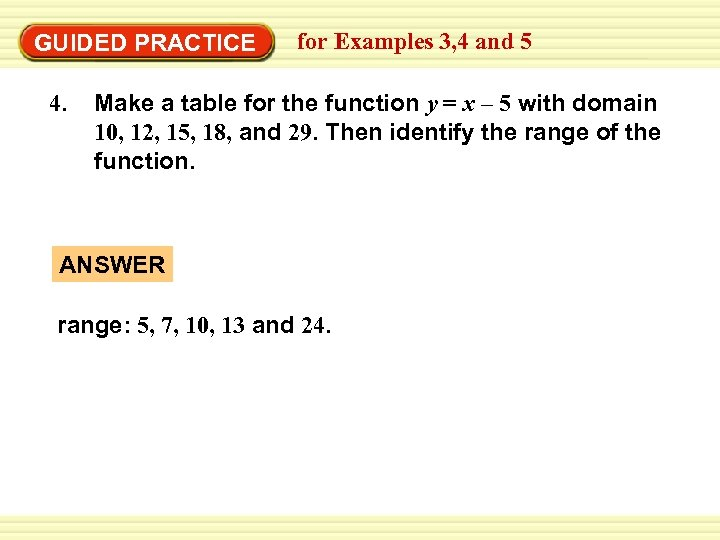GUIDED PRACTICE 4. for Examples 3, 4 and 5 Make a table for the function y = x – 5 with domain 10, 12, 15, 18, and 29. Then identify the range of the function. ANSWER range: 5, 7, 10, 13 and 24.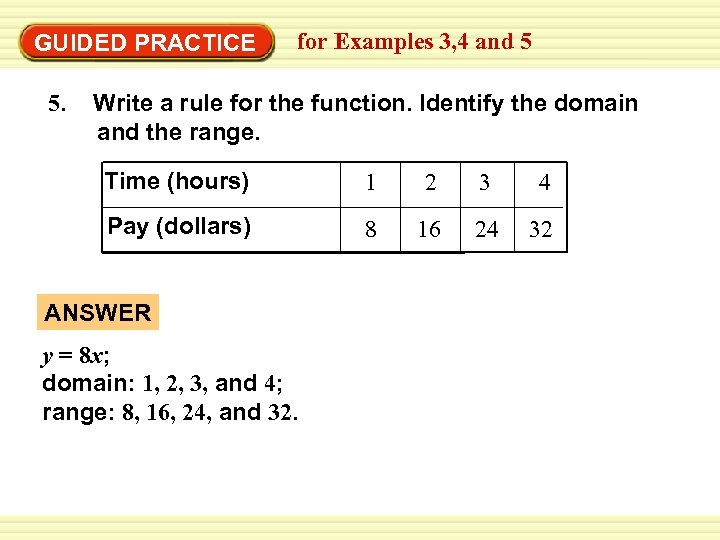GUIDED PRACTICE 5. for Examples 3, 4 and 5 Write a rule for the function. Identify the domain and the range. Time (hours) 1 2 3 4 Pay (dollars) 8 16 24 32 ANSWER y = 8 x; domain: 1, 2, 3, and 4; range: 8, 16, 24, and 32.